# SAT Math Multiple Choice Question 560: Answer and Explanation

### Test Information

Question: 560

5. In chemistry, the combined gas law formula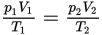gives the relationship between the volumes, temperatures, and pressures for two fixed amounts of gas. Which of the following gives p2 in terms of the other variables?

• A.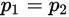• B.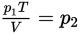• C.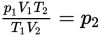• D.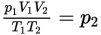Explanation:

C

Difficulty: Medium

Category: Passport to Advanced Math / Exponents

Strategic Advice: Don't spend too much time worrying about the scientific explanation of the equation. Focus on the question at the very end—it's just asking you to solve the equation for p2.

Getting to the Answer: Multiply both sides by T2 to get rid of the denominator on the right-hand side of the equation. Then divide by V2 to isolate p2.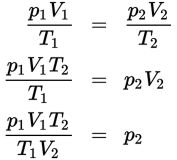Stop here! You cannot cancel the V's and T's because the subscripts indicate that they are not the same variable. In math, subscripts do not behave the same way superscripts (exponents) do.